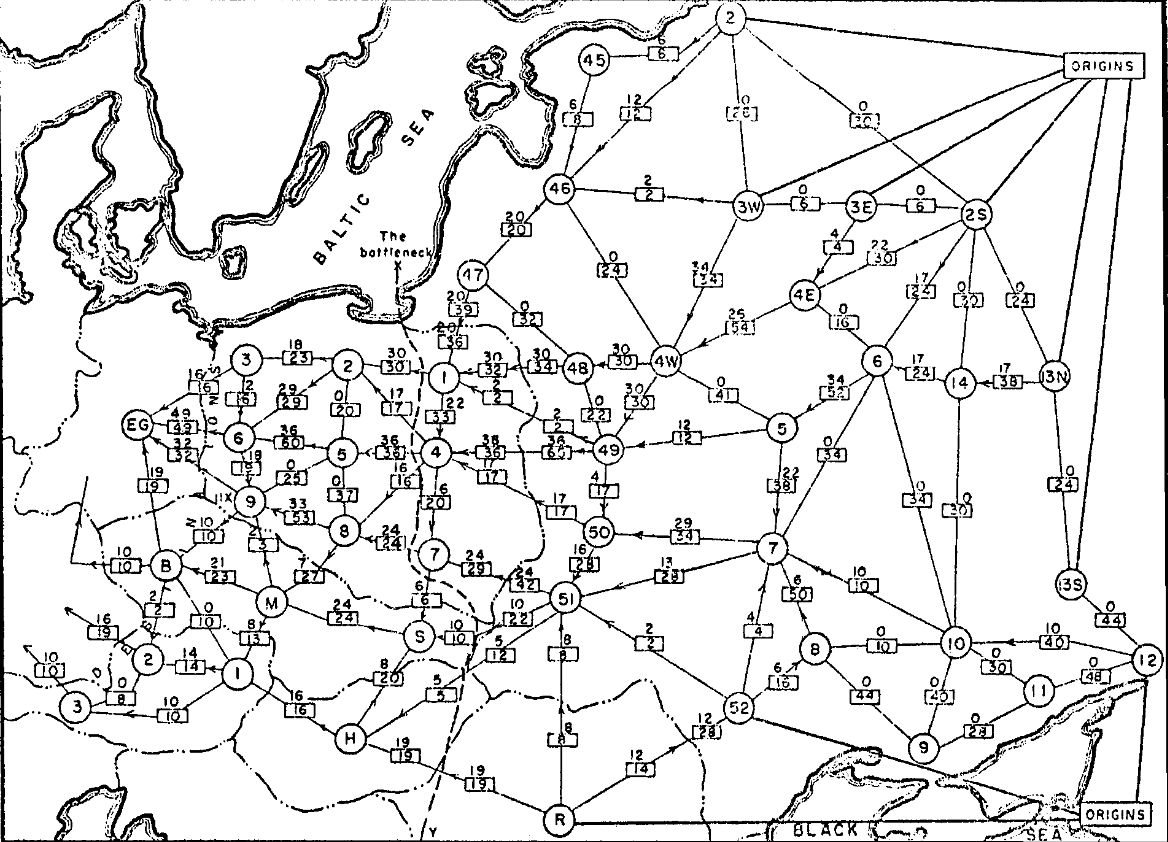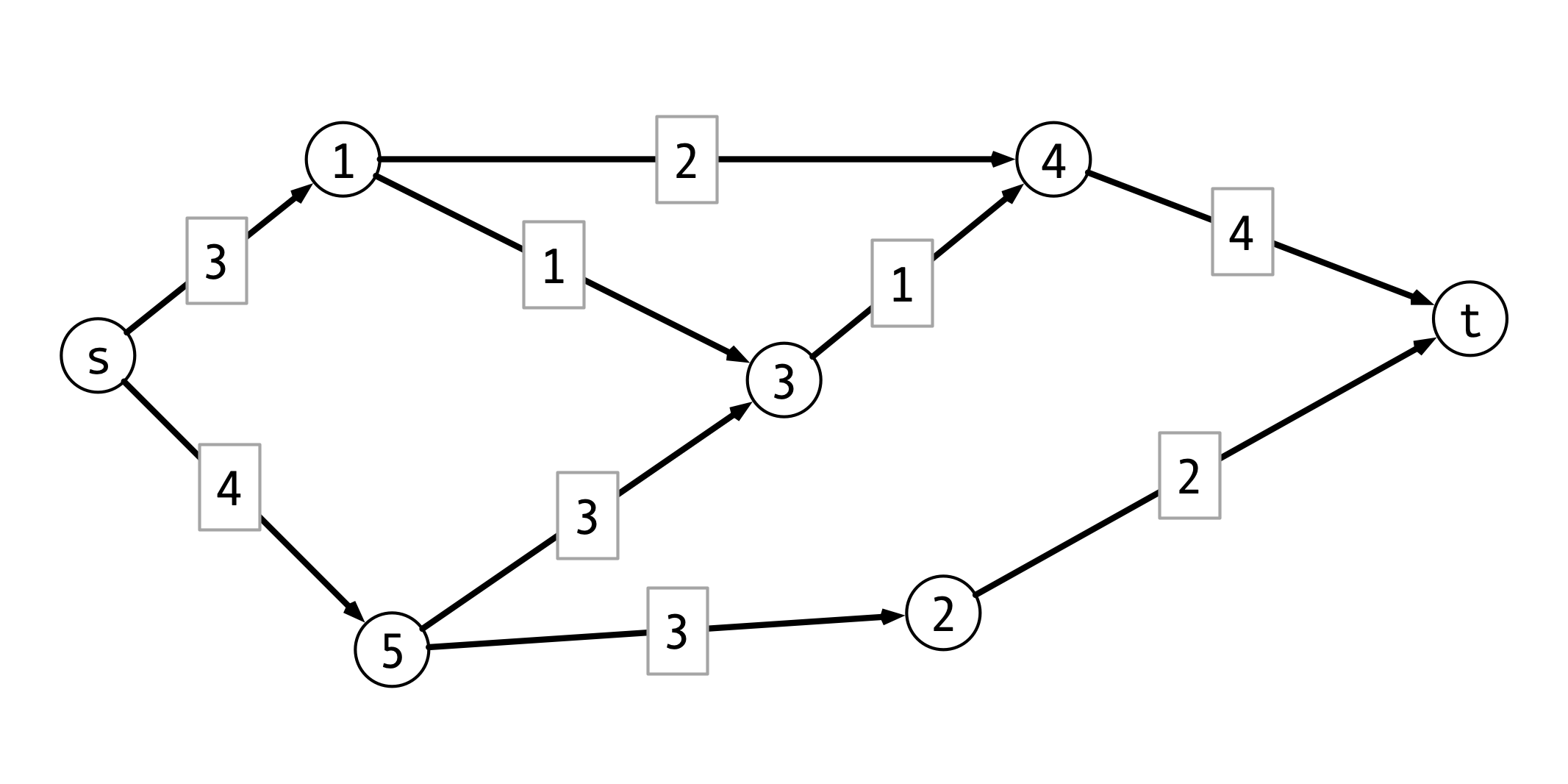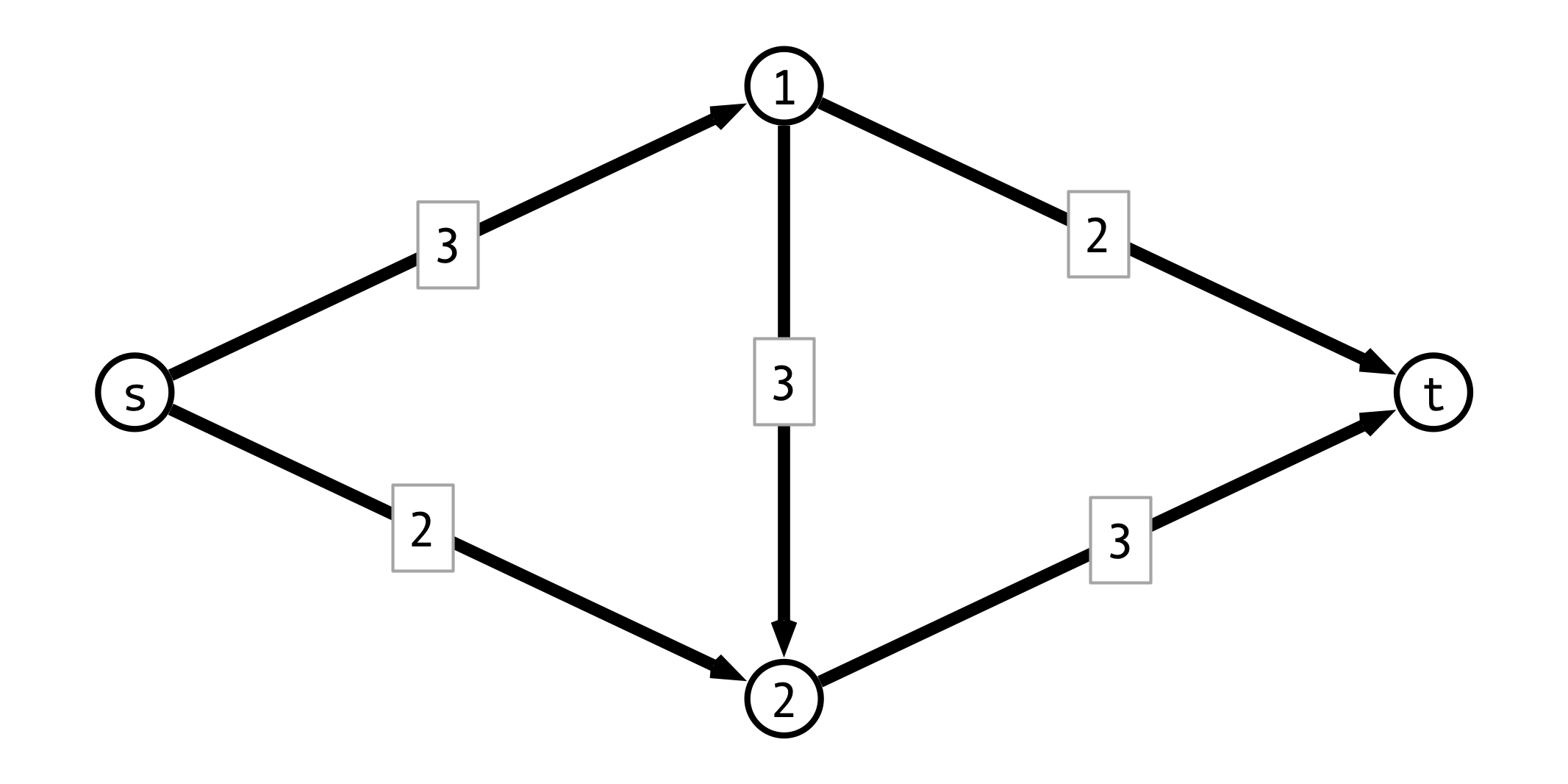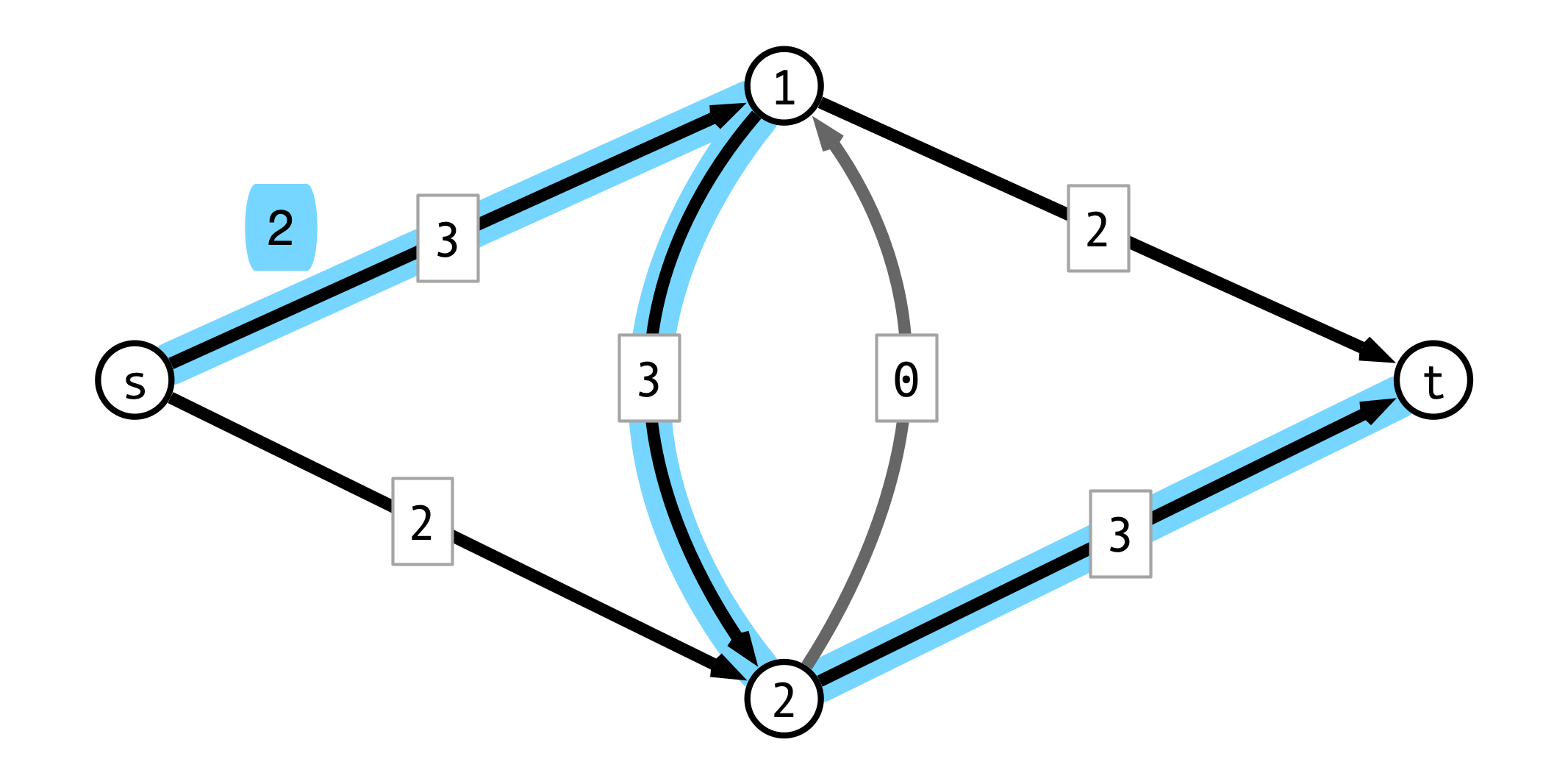# Lecture 28: Network Flow

$\def\opt{ {\mathrm{opt}} } \def\val{ {\mathrm{val}} }$

## Last Time

Bellman-Ford Algorithm for SSSP

Definition. For each $j = 0, 1, \ldots, n-1$ define $d_j(u, v) =$ length of shortest path from $u$ to $v$ consisting of $\leq j$ hops.

Observations.

1. If $G$ has no negative weight cycles then $d(u, v) = d_{n-1}(u, v)$
2. For all $j$, $d_j(u, x) = \min (d_{j-1}(u, x), \min_{v \to x} d_{j-1}(u, v) + w(v, x))$

Idea. Use second observation to compute $d_0(u, x), d_1(u, x), \ldots, d_{n-1}(u, x)$ for all $x$.

## Bellman-Ford Algorithm

  Bellman-Ford(V, E, w, u)
d <- 2d array [0..n-1, 1..n]
for v = 1 to n do d[0, v] <- infinity
d[0, u] <- 0
for j = 1 to n-1 do
for each vertex v in V set d[j, v] <- d[j-1,v]
for each vertex v in V
for each neighbor x of v
d[j, x] <- Min(d[j, x], d[j-1, v] + w[v, x])
return d[n-1]


Running time is $O(m n)$ if $G$ has $n$ vertices and $m$ edges.

## Correctness

Claim. For all $j = 0, 1, \ldots, n-1$ and for all vertices $v$, $d[j, v]$ stores length of shortest path from $u$ to $v$ with $j$ or fewer hops. I.e., $d[j, v] = d_j(v)$

Proof. Induction on $j$.

Base case, $j = 0$.

## Inductive Step, $j-1 \implies j$

• suppose $d[j-1, v] = d_{j-1}(v)$ for all $v$
• consider shortest path $P$ of $\leq j$ hops from $u$ to $v$
• let $x$ be penultimate vertex in $P$
• then $d_j(v) = d_{j-1}(x) + w(x, v)$
• by inductive hypothesis, $d_{j-1}(x) = d[j-1,x]$
• therefore in iteration $j$, get $d[j, v] \leq d[j-1,x] + w(x, v) = d_{j-1}(x) + w(x, v) = d_j(v)$
• also have $d[j, v] \geq d_j(v)$ (why?)
• so $d[j, v] = d_j(v)$

## Conclusion

If $G$ has no negative weight cycles, then Bellman-Ford solves single source shortest paths in $O(m n)$ time.

## Dijkstra vs Bellman-Ford?

Running times:

• Dijkstra: $O(m \log n)$
• Bellman-Ford: $O(m n)$

Why pick Bellman-Ford over Dijkstra?

• Why might Bellman-Ford be preferable even if graph has no negative weight edges?

## Bellman-Ford Again

  Bellman-Ford(V, E, w, u)
d <- 2d array [0..n-1, 1..n]
for v = 1 to n do d[0, v] <- infinity
d[0, u] <- 0
for j = 1 to n-1 do
for each vertex v in V set d[j, v] <- d[j-1,v]
for each vertex v in V
for each neighbor x of v
d[j, x] <- Min(d[j, x], d[j-1, v] + w[v, x])
return d[n-1]


# Cold War

## Soviet Rail Network, ca. 1955## Networks to Graphs

Modeling the network:

• nodes represent railway junctions
• edges represent rail lines
• weights represent capacities of lines
• capacity $=$ tonnage that can cross line per unit time
• proportional to cost of disrupting line

Question 1. How much material can the USSR transport to Western Europe per unit time?

Question 2. What is the cheapest way to disrupt flow of all material?

• Harris & Ross, 1955 USAF, declassified 1999

## Network Flow

A new interpretation of directed graphs:

• network of (directional) pipes
• weights are capacities
• how much fluid can flow through piper per time
• designated source node $s$
• all edges directed away from $s$
• designated sink or destination node $t$
• all edges directed towards $t$

Question. How much fluid be routed from $s$ to $t$ per unit time?

## Example## Flows, Formally

Setup.

• $G = (V, E)$ a directed graph, $s, t$ source and sink
• $c(u,v)$ is capacity of edge $(u, v)$

Flows. An s-t flow $f$ is a function $f : E \to \mathbf{R}^+$ satisfying:

1. capacity constraints: for each edge $e$, $f(e) \leq c(e)$
2. conservation: for every vertex $v \neq s, t$, flow into $v =$ flow out of $v$:
• $\sum_{x \to v} f(x, v) = \sum_{v \to y} f(v, y)$

The value of the flow $f$ is $\val(f) = \sum_{s \to v} f(s, v)$

## Max Flow Problem

Input.

• weighted directed graph $G = (V, E)$
• weights = edge capacities $> 0$
• source $s$, sink $t$
• all edges oriented out of $s$
• all edges oriented into $t$

Output.

• flow $f$ of maximum value
• $\val(f) = \sum_{s \to v} f(s, v)$

## A Simple Greedy Strategy

Repeat until done:

1. find an “unsaturated” path $P$ from $s$ to $t$
2. find minimum (remaining) capacity $b$ along $P$
3. route $b$ units of flow along $P$

## Greedy Approach Example## Choosing Different First Path## Greedy Issue

Flow along $P$ may block other viable paths

Question. How to fix this?

## Augmenting Paths

Idea. Add “undo” feature for each edge

• if $f$ routes $f(u, v) \leq c(u, v)$ flow from $u$ to $v$, add reverse edge $(v, u)$ with capacity $c(v, u) = f(u, v)$

• using $(v, u)$ corresponds to “pushing back” flow from $(u, v)$

• if an alternate route for this flow can be found, then more flow can be routed through $u$

## Pushing Back Example## The Residual Graph

• $G = (V, E)$ original graph
• $f$ a flow on $G$

Residual graph $G_f = (V_f, E_f)$

• vertex set $V_f = V$
• for each $(u, v) \in E$, add $(v, u)$ to $E_f$
• $(u, v)$ is forward edge
• $(v, u)$ is backward edge
• in $G_f$ capacity of $(u, v)$ is:
• $c(u, v) - f(u, v)$ if $(u, v) \in E$ (forward edge)
• $f(v, u)$ if $(v, u) \in E$ (backward edge)

## Residual Graph Example## Ford-Fulkerson Algorithm

Very high level

1. Initialize residual graph, flow $f$
2. While there is a path from $s$ to $t$ in residual graph do:
• find path $P$ from $s$ to $t$
• ignore edges with capacity $0$
• $b \leftarrow$ minimum capacity along $P$
• augment flow $f$ by $b$ along $P$
• update residual graph
3. return $f$

## Question

We’ve found a path $P$ with minimum capacity $b > 0$!

Question. How do we…

1. update flow $f$?
2. update residual graph $G_f$?

## Formalizing Ford-Fulkerson

  MaxFlow(G, s, t):
Gf <- G
f <- zero flow
P <- FindPath(Gf, s, t)
while P is not null do:
b <- min capacity of any edge in P
Augment(Gf, f, P, b)
P <- FindPath(Gf, s, t)
endwhile
return f


## Augment Procedure

  Augment(Gf, f, P, b):
for each edge (u, v) in P
if (u, v) is forward edge then
f(u, v) <- f(u, v) + b
c(u, v) <- c(u, v) - b
c(v, u) <- c(v, u) + b
else
f(v, u) <- f(v, u) - b
c(v, u) <- c(v, u) + b
c(u, v) <- c(u, v) - b


## Running Time

Assume:

1. all capacities are integers
2. $C =$ sum of capacites of edges out of $s$

Observe:

1. How long to find augmenting path $P$?

2. How long to run Augment?

3. How many iteraions of find/augment?

Conclude: Overall running time?

## Next Time

Ford-Fulkerson Correctness!